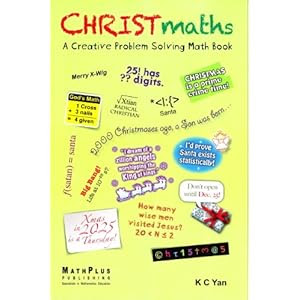## Welcome to K C Yan's Singapore Math blog!

Wanting to be updated on Singapore Math news or new Singapore Math? You have come at the right place! Please leave your comments before leaving. A googol thanks.

## Saturday, December 17, 2011

### 25 Christmaths Toughies from Singapore

Here are 25 non-routine fertile questions for friends and relatives to solve during this festive season:

1. The Recurring 25

Convert 0.252525... to a fraction.

2. The Sides of a Polygon

If the sum of the interior angles of a polygon is 2525°, what is the maximum number of sides the polygon can have?

3. December 25, 2025

On what day of the week does Christmas Day fall in the year 2025?

4. Number of Diagonals in a 25-gon

What is the number of diagonals in a 25-sided convex polygon?

5. How Many Ways?

In how ways can you arrange the letters of the word CHRISTMASTIME?

6. Merry Xmas & Happy New Year

If the value of MERRY CHRISTMAS is 189, the value of A HAPPY NEW YEAR is _____.

7. Santa's Routes

Determine the total number of distinct shortest routes from point A to point B in the following diagram grid map.
 A

 B

8. A Sum of 25 Powers

Find the sum of S = 2 + 21 + 22 + 23 +⋯+ 225.

9. Writing 25

What is another way of getting the number 25 using the numbers 2 and 5 only once, other than equating it to 5²?

10. Time Needed

Determine how long it will take to return all the gifts mentioned in the song “The twelve days of Christmas” if the gifts are returned at the rate of one gift per day.

11. Join the Xmases Family

A mother who was born on December 25, married to a husband also born on December 25, gives birth to their first baby on December 25. What are the odds of the mother doing that?

12. Four Fours to Make 25

Using four fours, the four basic operations and, if necessary, √4 and/or 4!, form the number 25. [Note: 4! = 1 × 2 × 3 × 4]

13. The Largest 25-digit Integer

What is the largest 25-digit number that can be divided by 2 and 5 without any remainder?

14. Number of Zeros in 1 × 2 × 3 ×⋯× 24 × 25

How many zeros are there at the end of the product 1 × 2 × 3 ×⋯× 24 × 25?

15. The Chocolate Bar

Given a 25-piece square chocolate bar, how many snaps are required to break the bar into its individual pieces?

16. A Creative Thinking Question

It is late on Christmas Eve and little Noel is waiting the Christmas tree to be finished. At exactly what time will this happen?

17. Christmas and Halloween

Prove that Oct. 31 = Dec. 25.

18. Divisibility by 25

Show that, if n is a positive integer, 16n + 10n – 1 is divisible by 25.

19. A Pair of Christmas Alphametics

In each case, replace each letter with a digit, different letters being different digits.

(a) SANTA - CLAUS = XMAS

(b) A + MERRY + XMAS = TURKEY

20. A Sum of Squares

Find the value of 1² – 2² + 3² – 4² +⋯+ 25².

21. Number of Rectangles

A board has 25 squares. How many individual rectangles are there in all?

22. A Christmas Party

At a Christmas party, each child brought a present. Presents were put in a large basket. All presents were different but identically wrapped. Going home, each child randomly selected a present from the basket. What is the expected number of children who carry home their own presents?

23. How Many Lines?

Using only horizontal and vertical lines, what is the most number of straight lines you can draw between the dots in a 5 × 5 grid without lifting your pencil?

24. A Table of 25 Boys and 25 Girls

A total of 25 boys and 25 girls sit around a table. Show that it is always possible to find a person both of whose neighbors are girls.

25. All n's End in Zeros

For what values of n does 1 × 2 × 3 ×⋯× (n – 1) × end in 25 zeros?

A Merry CHRISTmaths to all!

1. 25/99     2. 16 sides.         3. Monday.      4. 275 diagonals.     5. 778,377,600 ways.
6. 158.       7. 252 routes.      8. 2^26 - 2      9. 5 ÷ .2 = 25          10. 364 days.
11. 364/1         12. 4! + (√4 + √4)/4             13. 999…99990     14. 6.         15. 624 snaps.

16. Midnight.       18. 600.       19. (b) 2 + 97,445 + 6,928          20. 325.
21. 225 rectangles.                    22. 1.                 23. 34 lines.      25. 105, 106, 107, 108, 109

For full solutions, consult the title in the Reference.

Reference
Yan, K. C. (2011). CHRISTmaths: A Creative Problem Solving Math Book. Singapore: MathPlus Publishing.

© Yan Kow Cheong, Dec. 16, 2011.Now available as an iPad app http://tinyurl.com/7pn8pau